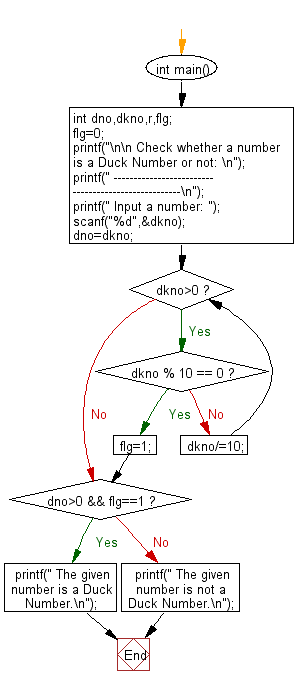﻿ C Program: Check whether a number is a Duck Number or not - w3resource# C Exercises: Check whether a number is a Duck Number or not

## C Numbers: Exercise-23 with Solution

Write a program in C to check whether a number is a Duck Number or not.

Test Data
Input a number: 3210

Sample Solution:

C Code:

``````# include <stdio.h>
# include <stdlib.h>

int main()
{
int dno,dkno,r,flg;
flg=0;
printf("\n\n Check whether a number is a Duck Number or not: \n");
printf(" ----------------------------------------------------\n");
printf(" Input a number: ");
scanf("%d",&dkno);
dno=dkno;
while(dkno>0)
{
if(dkno % 10 == 0)
{
flg=1;
break;
}
dkno/=10;
}
if(dno>0 && flg==1)
{
printf(" The given number is a Duck Number.\n");
}
else
{
printf(" The given number is not a Duck Number.\n");
}
}
```
```

Sample Output:

``` Input a number: 3210
The given number is a Duck Number.
```

Pictorial Presentation:Flowchart:C Programming Code Editor:

What is the difficulty level of this exercise?

Test your Programming skills with w3resource's quiz.

﻿

## C Programming: Tips of the Day

Why do C and C++ compilers allow array lengths in function signatures when they're never enforced?

It is a quirk of the syntax for passing arrays to functions.

Actually it is not possible to pass an array in C. If you write syntax that looks like it should pass the array, what actually happens is that a pointer to the first element of the array is passed instead.

Since the pointer does not include any length information, the contents of your [] in the function formal parameter list are actually ignored.

Ref : https://bit.ly/3fhlvdH